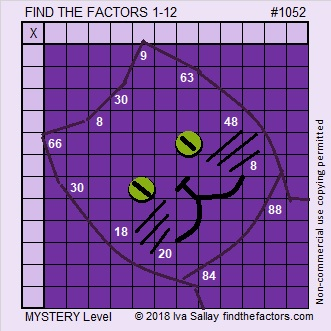1052 A Mysterious Purple Cat for Josephine

My very brilliant friend, Josephine likes cats and her favorite color is purple. Hence, this is a purple cat puzzle made especially for her. It’s a mystery level puzzle so its difficulty level is a big secret. This puzzle only requires skills in multiplication and division. I’m sure it will be no match for Josephine, who can easily handle more advanced mathematics such as calculus. Josephine is also fluent in English, Chinese, Spanish, French, Arabic, and Tajiki.  She is very busy, so hopefully, she’ll be able to find the time to spend some time with this mysterious purple cat.Print the puzzles or type the solution in this excel file: 12 factors 1044-1053

If the colors in the puzzle distract you, here is the same puzzle in very plain black and white:Here is some information about the number 1052:

Its last two digits are 52, so it is divisible by 4.

• 1052 is a composite number.
• Prime factorization: 1052 = 2 × 2 × 263, which can be written 1052 = 2² × 263
• The exponents in the prime factorization are 2 and 1. Adding one to each and multiplying we get (2 + 1)(1 + 1) = 3 × 2  = 6. Therefore 1052 has exactly 6 factors.
• Factors of 1052: 1, 2, 4, 263, 526, 1052
• Factor pairs: 1052 = 1 × 1052, 2 × 526, or 4 × 263
• Taking the factor pair with the largest square number factor, we get √1052 = (√4)(√263) = 2√263 ≈ 32.4345494804537095484851052 is palindrome 282 in BASE 21 because 2(21²) + 8(21) + 2(1) = 1052